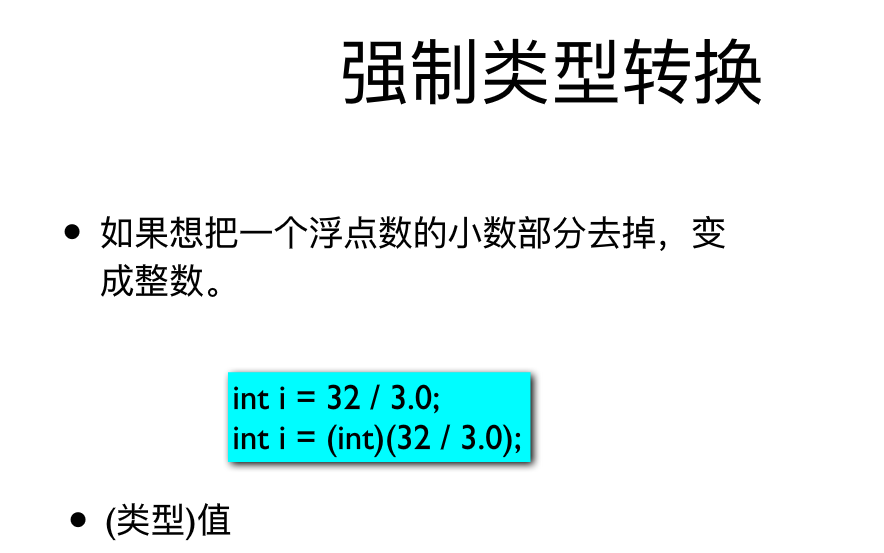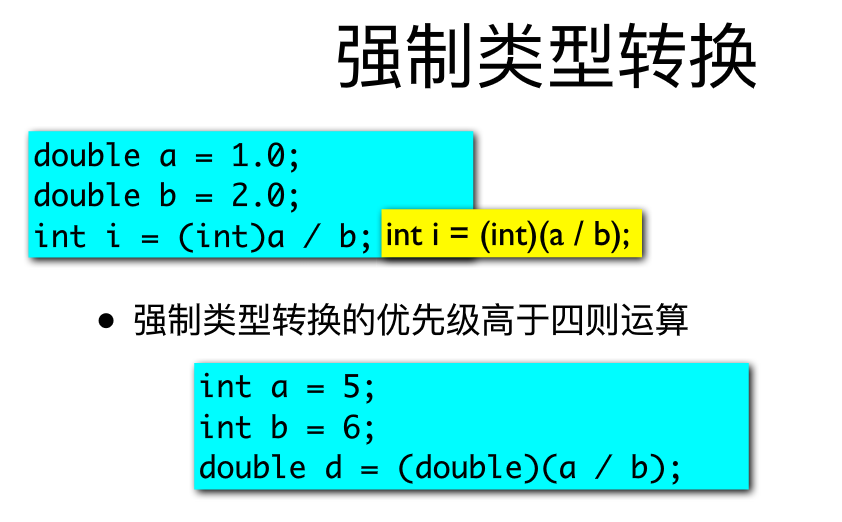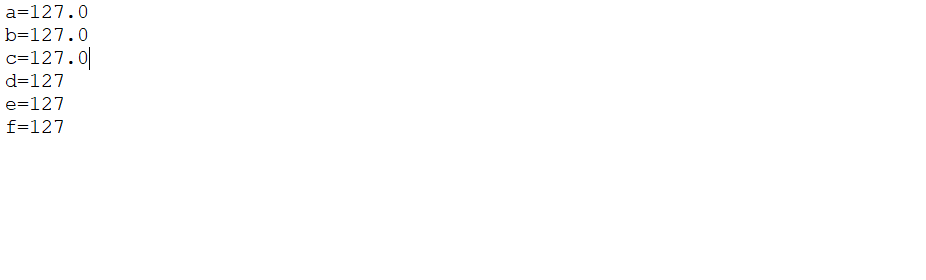• 将numpy array由浮点型转换为整型，使用numpy中的astype()方法可以实现。
使用numpy中的astype()方法可以实现，示例如下：

x
Out:
array([[ 5.        ,  4.        ],
[ 4.        ,  4.33333333],
[ 3.66666667,  4.5       ]])

x.astype(int)
Out:
array([[5, 4],
[4, 4],
[3, 4]])

参考：http://stackoverflow.com/questions/10873824/how-to-convert-2d-float-numpy-array-to-2d-int-numpy-array
展开全文numpy array
• public class Basic{ public static void main(String[] args){ double a = 10.0; double b = 3.0; System.out.println("a / b = " + (int)(a /...利用(int)(非int类型结果的表达式或值) 转为int。
public class Basic{
public static void main(String[] args){
double a = 10.0;
double b = 3.0;
System.out.println("a / b = " + (int)(a / b));
}
}
输出
a / b = 3
利用(int)(非int类型结果的表达式或值) 转为int型。展开全文• 在c/c++中我们经常由于需要，要把浮点型数据（float、double）转换成整形数据（各种int），下面我给大家介绍一下我所知道的，希望能给大家以后的编程带来方便： 1.普通的强制转换：可实现 “下行”整型化——即将...
 在c/c++中我们经常由于需要，要把浮点型数据（float、double）转换成整形数据（各种int），下面我给大家介绍一下我所知道的，希望能给大家以后的编程带来方便：
1.普通的强制转换：可实现 “下行”整型化——即将浮点数变成小于它的最大整数
c标准     int i;
double x=5.4;
i=(int)x;
此时我们得到的i的值为5；即将浮点型的小数部分去掉。
c++标准   int i;
double x=5.4;
i=int(x);
所得结果与前者一样，i的值为5，即将浮点型的小数部分去掉。
2.实现四舍五入（m舍n入）：
想几舍几入最简单的办法是在原数上加个0.n就可以了，如：想四舍五入就——5.4  int(5.4+0.5)=5
5.5   int(5.5+0.5)=6
又如：想二舍三入就——5.2  int(5.2+0.7)=5                                                                                                                         5.3 int(5.3+0.7)=6
3.实现“上行”整型化：即
①使用c/c++标准库函数：ceil()  需要加载头文件c中加载#include<math.h>  c++中加载#include<cmath>
具体如：int i;      double x=5.4;        i=ceil(x);      // i 等于6
int i;       double=2.0;          i=ceil(x);      // i 等于2
②自己编写函数，实现“上行”整型化：即将浮点数转换成大于它的最小的整数。
举例—if(x-(int)x)  i=(int)x+1;   //如果 x-(int)x不等于0（这里实际上是 大于0），则 i 等于 i 的“下行”整型化加1
else i=(int)x;           //否则（即x-(int)x=0) i 等于（int）i   这里表面上是进行了“下行”运算，实际上
x=n.0    （int) x 就是将 2.0 变成 2  。
展开全文• 在Java中，我们如何将整型转化为浮点型，或者我们如何将浮点型转化成整型的呢？ 结果演示 代码演示 package com.ten; public class Zidongzh { public static void main(String[] args) { double a = 127.0; ...
在Java中，我们如何将整型转化为浮点型，或者我们如何将浮点型转化成整型的呢？
结果演示代码演示
package com.ten;

public class Zidongzh {
public static void main(String[] args) {
double a = 127.0;
float b=(float)a;
float c=(float)b;
int d=(int)c;
short e=(short)d;
byte f=(byte)e;

System.out.println("a="+a);
System.out.println("b="+b);
System.out.println("c="+c);
System.out.println("d="+d);
System.out.println("e="+e);
System.out.println("f="+f);
}

}


展开全文• ## 浮点型转整型

千次阅读 2019-02-26 08:46:02
今天朋友编一个程序遇到要把浮点型数据转换为整型需要把小数部分进一。比如1.234经转换等于2。 我查了很多地方都没有很好的解答。终于发现int函数与fix函数在转换中的区别，当数据正时他俩没区别，但当数据负...vb.net integer math c
• [注明] 现有的Intel中的SSE指令 cvtps2dq XMM,XMM/m128　支持把源存储器4个单精度浮点数变成4个双字有符号整数,结果送入目的寄存器,内存变量必须对齐内存16字节，另外还有其他指令支持双精度和整型转换。...c语言 浮点数
• 字符串，整型浮点型等类型之间的转换可以举一反三，此处举例将浮点型转为整型的例子： 下面展示一些 内联代码片。 1. map(int, list) a = [0.0567, 9.2345, 8.1986, 4.3333]; a_int = list(map(int, a)) 2.for ...
• 方便的把浮点型转换为字节，也可以把字节转换为浮点型；另外还支持I32与十进制相互转换。如果杀毒软件报错，请添加信任即可。
• 1.应用场景 数据类型显式强制转换，比如接收参数时，【后端/php】容错写法 //前端参数约束... //类型转换 将字符串转换为整型浮点型 function conversionStringToIntOrFloat($data){ foreach($data as \$...字符串
• include <stdio.h> int main() { double d; scanf("%lf", &d); int a = (int)d; //正 if (d >= 0) { if (d + 0.5 >= a + 1.0) printf("%d", a + 1); else printf("%d", a);... //...c语言 开发语言 后端
• 主要是强制类型转换部分，用常用的devcpp测试是向零取整。 想知道是所有都是向零取整还是会根据编程环境不同而不同？有问必答 c++
• 1. 浮点数据较大，很少有数据小于0.5，这样可以直接转为整型，不需要扩大像素。 打开ArcToolBox工具，选择Spatial Analysis工具，数学分析，转为整型 输入相应的数据 点击确定。 2. 浮点数据较小，像素大小多为...栅格数据处理
• 当需要将没有属性表的浮点数据转换为矢量数据时，在使用Raster to Polygon时，会无法读取栅格数据，这时可以尝试将浮点型的栅格数据转换为整型，或许可以解决该问题。这一步骤可以使用以下工具： Spatial Analyst ...大数据
• 函数将接收到的变量转换成实数（浮点型 float；整型int）储存在（arrays）数组中，数组默认保存三个实数（可更改）。 二，函数代码 /** *函数功能 ：接收到的数据转换浮点型 *rx ：单次接收到的字符 *a字符串 stm32 串口通信
• } IEEE浮点数的存放格式 对于大小32-bit的浮点数(32-bit单精度，64-bit浮点数双精度，80-bit扩展精度浮点数)，1、其第31 bit符号位，0则表示正数，反之复数，其读值用s表示; 2、第30～23 bit...c语言
• 字符串与整型浮点型数据之间的转换PAT 1054(求平均值)题解类型转换函数字符串转化其他类型其他类型转化字符串       最近遇到的一道题。其中用到了将字符串转化float类型，在...
• 最近做嵌入式项目，把一段老代码一直到智能手机平台之后发现...简单来说就是一个负的FLOAT强转为WORD会变成0，比如FLOAT f = -1.0;WORD w = f;w会变成0，而不是期待的0xffff（65535）。于是我在PC上用devc++（win版g语言 c 汇编 gcc behavior
• ，你可以先转换为float，然后转换为整数： >>> int('5') 5 >>> float('5.0') 5.0 >>> float('5') 5.0 >>> int(5.0) 5 >>> float(5) 5.0 >>> int('5.0') Traceback (most recent call last): File "<stdin>", ...
• 在32位的机器中，第一位表示符号位，第二位到底9位表示指数位 剩下的位表示小数位。 给出代码 // 1021.1.cpp : 定义控制台应用程序的入口点。 // #include "stdafx.h" #include typedef unsigned char * byte_...浮点数
• 将变量i的类型强制转换为浮点型，并将其值赋给变量a 将变量j的类型强制转换为整型，并将其值赋给变量b
• ... #include using ...强制转换为int后是5； 解决方案是： 不要将double的数据赋给整型，否则可能出现与初衷不符。   转载于:https://www.cnblogs.com/xiaochou/p/double2int.html
• 1、将一个字符串转换整型（map和reduce） from functools import reduce def str2int(s): def char2int(ch): c = {str(x): x for x in range(10)} return c[ch] def fun(n1, n2): return n1 * ...
• 参考文章链接：1.http://c.biancheng.net/cpp/html/1573.html2.http://c.biancheng.net/cpp/html/1574.html1.整型浮点型——&gt;字符串整数转换为字符串：--------------------------------------------------...C语言 数字 字符串
• class java3_2 { public static void main(String... 以下三种写法都可以使运算结果浮点数： （float）a/b a/(float)b (float)a/(float)b 要使结果浮点数，就必须将两个整数种的一个或者二个强制转换为浮点数类型。java
• pycharm中字符串内容为浮点型时无法直接转换为整型。 如图： 应当将a转化为浮点型再转化为整型。 反之字符串内容为整型，可直接转为浮点型 本文初学者学习笔记，如有错误望大佬指出，内容具体原因还望大佬指点，...python
• 1.python 字符串和整数的转换 常用的格式化字符串： %d 整数 %f%F 浮点数 %e%E 科学计数 %g%G e 和%f/%E 和%F 的简写 %% 输出% 格式化操作符辅助指令 符号 作用 * 定义宽度或者小数点精度 - 用做左对齐 + 在...
• 我想写一个计算平均的函数，但计算结果总是不对。 program： float average(int* n[]) { int i; int sum = 0; float average; for (i = 0; i < 10; i++) { sum = sum + n[i]; } average =...c语言
• 两种不同的浮点型转为整型的方法； import java.math.*; public class Test { public static void main(String[] args) { double x = 9.6666666; int q = (int)x; int p = (int)Math.round(x); ...Java学习
• //filename: zhuanhuan.java public class zhuanhuan { public static void main(String[] args) { int a=155; int b=9; float g,h; System.out.println("a="+a+",b="+b); g=a/b; System.out.println("a/b="+g+...Syst...